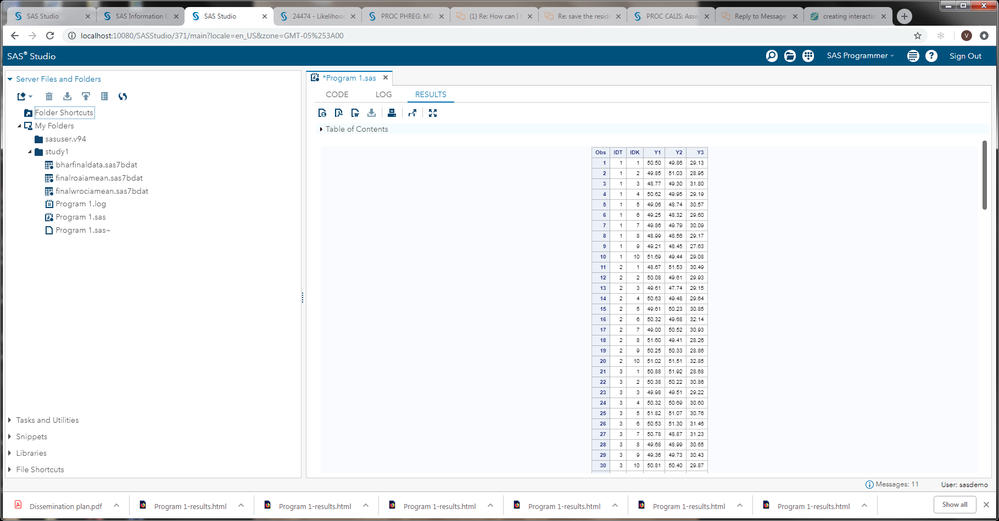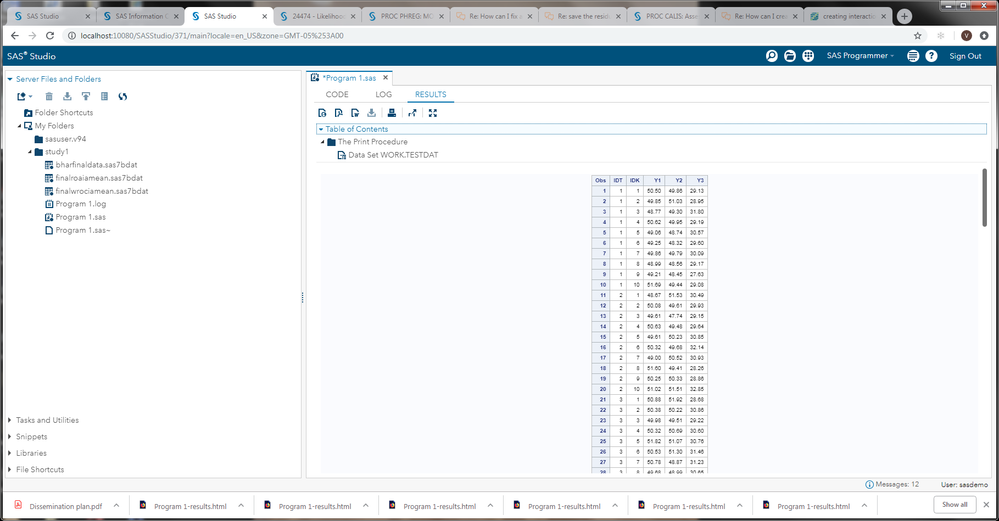## How can I create the correct number of students?

There was also an issue with the code by creating the correct number of students in the sample. It was only creating 10 students total, but I believe it needs to create 10 students per teacher. Each teacher cannot have the same 10 students, they each need their own set of students. For example, teacher ID1 has 10 students. Teacher ID2 needs to have 10 students who are different from 10 students of teacher ID1. Teacher ID3 needs to have  10 students who are different from 10 students of teacher ID1/ID2. There are 15 teachers. We need to create 10 students per teacher. Thus, the total number is 150 (15*10). I used the following code in bold. But it was creating 10 students total. For example, teacher ID 1 has 10 students. Teacher ID2 has 10 students who are the same as 10 students of teacher ID1. Teacher ID 3 has 10 students who are the same as 10 students of teacher ID1/ID2. Thus, it is wrong. How can I fix the code?

`/*Create the number of students in the sample*/%let nt = 15; /*number of teachers*/%let nb = 10; /*number of students*/%let n1=3; /*number of outcomes*/%let nanb = &nt*&nb;/*teacher cov - this a cov matrix from the corr matrix*/%let tcov = {.25 .175 .0225, .175 .25 .0225, .0225 .0225 .0625};/*student cov*/%let scov = {1 .2 .0225, .2 1 .0225, .0225 .0225 1};                      /*mean vector*/%let mv= {0,0,0};proc iml;gmv={50 50 30}; /*grand mean vector*/	ynew=j(&nanb,&n1,0); /*this is for the new outcomes, should be as long as the stu*tch*/		y=j(&nb,&n1,0); 			tch_res=j(&nt,&n1,0);				stu_res=j(&nb,&n1,0);call randseed(4321589);      do A = 1 to &nt;      tch_res[A,]= (RandNormal(1,&mv,&tcov)); /* tchres matrix*/          do B = 1 to &nb;           stu_res[B,]= (RandNormal(1,&mv,&scov)); /*stures matrix*/				do C = 1 to &n1;			if C = 1 then do; 			y[B,1] = gmv[1,1] + tch_res[A,1] + stu_res[B,1]; 			end;				if C = 2 then do;				y[B,2] = gmv[1,2] + tch_res[A,1] + stu_res[B,2]; 				end;					if C = 3 then do;					y[B,3] = gmv[1,3] + tch_res[A,1] + stu_res[B,3]; 					end;				end;		     end;	     yy = round(y,.01);            tn = &nb*(A-1)+1;       tend = &nb*A;	  ynew[(tn:tend)`,1:&n1] = yy;      y=J(&nb,&n1,0);end;id1 = (1:&nb)`; *print id1;idk = repeat(id1,&nt); *print idk;id2 = (1:&nt)`;idt = repeat(id2,&nb); *print id21;call sort(idt,1); *print idt;/*save tch resids and stu resids in files*/tchres=  tch_res;stures=  stu_res;create tchresids from tchres;append from tchres;close tchresids;create sturesids from stures;append from stures;close sturesids;  ydone = idt||idk||ynew; /*create data set to use in proc mixed*/c = {idt idk y1 y2 y3};create testdat from ydone [colname=c];  append from ydone;  close testdat;quit;/*proc print data=testdat (obs=100); run; */proc sort data=testdat;   by idt;run;proc surveyselect data=testdat    out=want sampsize=10;;    strata idt;run;data newdat;	set want; y=y1; wave=1; cwave=wave; output;y=y2; wave=2; cwave=wave; output;y=y3; wave=3; cwave=wave; output;	 keep idt idk y wave cwave;run;proc print data=newdat width=uniform; run;/*run the multivariate multilevel model*/proc mixed data=newdat covtest;   class idt idk cwave;   model y = cwave  / noint s residual outp=Resid;    random cwave / sub=idt type=un g gcorr solution;   repeated cwave / sub=idk(idt) type=un r rcorr;    ods output covparms=cp fitstatistics=fit;run;run;/*calc icc from covparm table*/data cparms;set cp;if subject = ' ' then subject = 'resid';run;proc transpose data=cp out=cp_trans;var estimate;id covparm subject;run;/* icc*/data cp;set cp_trans;iccT1 = (UN_1_1_IDT)/(UN_1_1_IDT + UN_1_1_IDK_IDT_);iccS1 = (UN_1_1_IDK_IDT_)/(UN_1_1_IDT + UN_1_1_IDK_IDT_);iccT2 = (UN_2_2_IDT)/(UN_2_2_IDT + UN_2_2_IDK_IDT_);iccS2 = (UN_2_2_IDK_IDT_)/(UN_2_2_IDT + UN_2_2_IDK_IDT_);iccT3 = (UN_3_3_IDT)/(UN_3_3_IDT + UN_3_3_IDK_IDT_);iccS3 = (UN_3_3_IDK_IDT_)/(UN_3_3_IDT + UN_3_3_IDK_IDT_);run; /*data generation check*/ PROC univariate data=testdat;var y1 y2 y3;run;  PROC corr data=testdat cov;var y1 y2 y3;run;  PROC univariate data=tchresids;var COL1 COL2 COL3;run;PROC univariate data=sturesids;var COL1 COL2 COL3;run;/*Apply independent univariate models*//*Math*/proc mixed data=testdat covtest;   class idt idk;   model y1 =  / s residual outp=mathresid;   random intercept / sub=idt type=un solution;   random intercept / sub=idk(idt) type=un solution;    ods output covparms=cpy1 fitstatistics=fity1;run;proc transpose data=cpy1 out=cpy1_trans;var estimate;id covparm subject;run;/*Read*/proc mixed data=testdat covtest;   class idt idk;   model y2 =  / s residual outp=readresid;   random intercept / sub=idt type=vc solution;   random intercept / sub=idk(idt) type=vc solution;    ods output covparms=cpy2 fitstatistics=fity2;run;/*Motivation*/proc mixed data=testdat covtest;   class idt idk;   model y3 =  / s residual outp=motresid;   random intercept / sub=idt type=vc solution;   random intercept / sub=idk(idt) type=vc solution;    ods output covparms=cpy3 fitstatistics=fity3;run;/RQ1//*Absolute bias, sum of difference between teacher level residual  in tch_res from the resdiuals from MM model and the uniM modelssum of(udj - uhatdj) divided by N*//*change predresid file to break out residuals by outcome*//*predresid are from the MM model*/run;PROC sort data=Resid;by cwave;run;Data MMresids (keep=idt idk cwave MMmathresid MMreadresid MMmotresid);set Resid;if cwave='1' then MMmathresid=Resid;if cwave='2' then MMreadresid=Resid;if cwave='3' then MMmotresid=Resid;run;proc sort data=MMresids;by idt idk;run;Data MMreadresid;set MMresids;if cwave='2';run; Data MMmathresid;set MMresids;if cwave='1';run;Data MMmotresid;set MMresids;if cwave='3';run;Data MMresids1;merge MMreadresid (keep=MMreadresid) MMmathresid (keep=MMmathresid) MMmotresid (keep=MMmotresid);run;/*create a new file with the residuals*/Data Residuals;Merge MMresids1 mathresid (rename=(resid=mathUtchres) keep=resid idk idt) readresid (rename=(resid=readUtchres) keep=resid) motresid (rename=(resid=motUtchres) keep=resid); run; Data Bias; /*this is the new file, bias is the sum of the difference for all teachers*/set Residuals; /*this is the file with the data*/Readdif= abs(MMreadresid - readUtchres);Mathdif= abs(MMmathresid - mathUtchres);Motdif = abs(MMmotresid - motUtchres);run;proc summary Data=Bias;var  Readdif Mathdif Motdif;output out=Biassum sum=;output out=Bias1 mean=;run;/* AVERAGE BIAS, sum of all the bias across 1000 replications, for each udj, divided by 1000*/Data AveBias;set Bias1;bias=sum(of bias1-bias1000); averagebias=(sum_range)/1000;run;PROC corr data=Bias;var MMreadresid readUtchres;run;PROC corr data=Bias;var MMmathresid mathUtchres;run;PROC corr data=Bias;var MMmotresid motUtchres;run;/*calculate SRMR*/PROC CALIS data=Bias; METHOD=ML / standardized root mean square residual OUTRAM=SRMR;run; `

8 REPLIES 8

## Re: How can I create the correct number of students?

You have some PRINT statements in your IML code that have been commented out. Un-comment them and run the program again. Do the expected number of observations get printed? Does any of these prints lead you to a better understanding of where the issue might be? Does the output data set from IML named TESTDAT have the desired number of observations?

--
Paige Miller

## Re: How can I create the correct number of students?

I ran the following code. And I got the expected number of observations (150 observations).

Y1, Y2, and Y3 for each ID seem to be different. Does it mean that each teacher has the different 10 students, and they each have their own set of students? But each teacher ID has the same student ID. Does it make sense?

You can see the data below:

`proc print data=testdat (obs=150); run; `

## Re: How can I create the correct number of students?

@eunkum wrote:

I ran the following code. And I got the expected number of observations (150 observations).

Y1, Y2, and Y3 for each ID seem to be different. Does it mean that each teacher has the different 10 students, and they each have their own set of students? But each teacher ID has the same student ID. Does it make sense?

You can see the data below:

`proc print data=testdat (obs=150); run; `

How can we see that data? It references a file on your computers hard drive.

## Re: How can I create the correct number of students?## Re: How can I create the correct number of students?

But YOU can see the data, what does it tell you when you look at it?

Also, you ask "Does it mean that each teacher has the different 10 students, and they each have their own set of students? But each teacher ID has the same student ID." Is IDK the student ID? If you look at your code, you are assigning numbers to IDK in such a way that each teacher will have students numbered 1 through 10.

``````id1 = (1:&nb)`; *print id1;
idk = repeat(id1,&nt); *print idk;``````

So you are getting what you asked for here. I don't know if that's what you want or not.

--
Paige Miller

## Re: How can I create the correct number of students?

When I look at the data, each teacher has the same 10 students. I want to have the students have unique IDs.How can I have student ID1-student ID150, and have 10 students per each teacher (teacher ID 1-15) at the same time?

## Re: How can I create the correct number of students?

@eunkum wrote:

I ran the following code. And I got the expected number of observations (150 observations).

`proc print data=testdat (obs=150); run; `

Yes, if you run this code, you will see 150 observations, because you are using OBS=150. So I'm not sure there is any value in your comment that there are 150 observations. If you take out OBS=150, then how many observations are there?

Y1, Y2, and Y3 for each ID seem to be different. Does it mean that each teacher has the different 10 students, and they each have their own set of students? But each teacher ID has the same student ID. Does it make sense?

You can see the data below:

Actually, I cannot see the data. But YOU can see the data, what does it tell you when you look at it?

--
Paige Miller

## Re: How can I create the correct number of students?

When I took out OBS=150, there are 150 observations. Here is the data.From The DO Loop